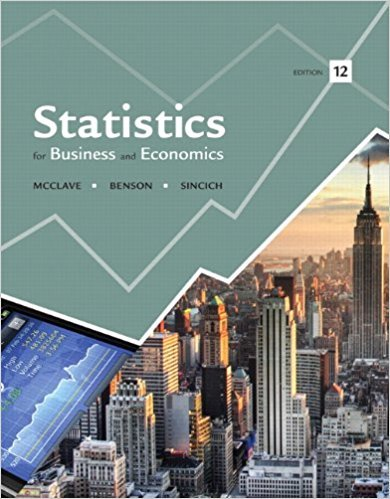×
Get Full Access to Statistics For Business And Economics - 12 Edition - Chapter 4 - Problem 105e
Get Full Access to Statistics For Business And Economics - 12 Edition - Chapter 4 - Problem 105e

×

# Optimal goal target in soccer. When attempting to score aISBN: 9780321826237 51

## Solution for problem 105E Chapter 4

Statistics for Business and Economics | 12th Edition

• Textbook Solutions
• 2901 Step-by-step solutions solved by professors and subject experts
• Get 24/7 help from StudySoup virtual teaching assistantsStatistics for Business and Economics | 12th Edition

4 5 1 303 Reviews
16
1
Problem 105E

Problem 105E

Optimal goal target in soccer. When attempting to score a goal in soccer, where should you aim your shot? Should you aim for a goalpost (as some soccer coaches teach), the middle of the goal, or some other target? To answer these questions, Chance (Fall 2009) utilized the normal probability distribution. Suppose the accuracy x of a professional soccer player’s shots follows a normal distribution with a mean of zero feet and a standard deviation of 3 feet. (For example, if the player hits his target, x = 0 ; if he misses his target by 2 feet to the right, x = 2 ; and if he misses 1 foot to the left, x = -1 .) Now, a regulation soccer goal is 24 feet wide. Assume that a goalkeeper will stop (save) all shots within 9 feet of where he is standing; all other shots on goal will score. Consider a goalkeeper who stands in the middle of the goal.

a. If the player aims for the right goalpost, what is the probability that he will score?

b. If the player aims for the center of the goal, what is the probability that he will score?

c. If the player aims for halfway between the right goalpost and the outer limit of the goalkeeper’s reach, what is the probability that he will score?

Step-by-Step Solution:

Step 1 of 3

(a)

Suppose the accuracyof a professional soccer player’s shots follows a normal distribution with a mean ofand a standard deviation of(For example, if the player hits his target, x = 0 ; if he misses his target by 2 feet to the right, x = 2 ; and if he misses 1 foot to the left, x = -1 .)

Now, a regulation soccer goal is 24 feet wide. Assume that a goalkeeper will stop all shots within 9 feet of where he is standing; all other shots on goal will score. Consider a goalkeeper who stands in the middle of the goal.

we are asked to find the probability that he will score if the player aims for the right goalpost.

If the goalkeeper stands in the middle of the goal and can any ball withinthen the only way a player can score if and only if he shoots the ball within theof either goal post.

If a player aims at the right goal post, then the player will score ifis betweenHence we need to find theThe probabilities for all normal distributions are computed by using the standard normal distribution. we can use the standard normal probability table and the appropriate

z values to find the desired probabilities.

The formula used to convert any normal random variable x with mean μ and standard deviation σ to the standard normal random variable z follows.We can rewriteas,Therefore, theforis,[meanand standard deviation]

Hence the area to the left ofis[Please refer the standard normal table II in Appendix]theforis,Hence the area to the left ofisHence the probability that he will score  if the player aims for the right goalpost isStep 2 of 3

Step 3 of 3

##### ISBN: 9780321826237

Unlock Textbook Solution